# [PL10] Derivations 1

Now you will learn a natural deduction system for MPL.

## §1. The rules are very similar

We adopt versions of the 12 rules from the natural deduction system for SL. While the old rules applied to SL formulas, these adopted rules apply to MPL formulas. Otherwise, the rules are unchanged. This provides rules for all the connectives of MPL.

Additionally, rules for the quantifiers '∃' and '∀' are needed. For each quantifier, there is an elimination rule and an introduction rule. With the four quantifier rules, that makes 16 rules in all.

Here are all of the rules, if you are in a hurry.

Explain why rule &E for MPL is a sound rule.

## §2. Rule of Existential Quantifier Introduction

The first quantifier rule is the rule of Existential Quantifier Introduction. Here is this rule in action to show Sa ⊢ ∃xSx:Rule ∃I says that you can take a formula you have written already, replace one or more occurrences of some name in the formula with a new variable, and then attach the existential quantifier to the front. The dependencies of the new formula are the same as the old formula.

Notice that ∃I is a sound rule. That means that when you use the rule, the formula you write down is entailed by its dependencies. Consider the previous example, where the rule is used at line 2. Note that for any interpretation under which "Sa" is true, "∃xSx" is true under that interpretation too.

Explain why for any interpretation under which "Sa" is true, "∃xSx" is true too.

Here is another example of ∃I in action: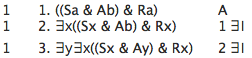Two details should be noted in using ∃I. The first detail is that ∃I applies to the whole formula, not to part of the formula. That means that the quantifier is added at the beginning of the formula, not in the middle. Thus, for example, the following derivation is incorrect: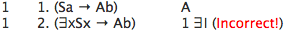If you could do this, then rule ∃I would not be sound because you could write down a formula which is not entailed by its dependencies. Line 2 is not entailed by its dependency, line 1. Take an interpretation under which "Sa" and "Ab" are false, but "Sb" is true. This is an interpretation under which "(Sa → Ab)" is true and "(∃xSx → Ab)" is false. So "(Sa → Ab)" does not entail "(∃xSx → Ab)".

So, remember: when using ∃I, the existential quantifier must be added to the front of the formula. The following is an example of a similar, but correct derivation: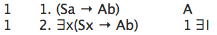Show (Fa & Ga) ⊢ (∃xFx & Ga)

The second detail to be noted about ∃I is that the formula you write down must be a well-formed formula of MPL. Thus, for example, the following derivation is not correct:The derivation is not correct because the formula on line 2 is not a well-formed formula of MPL. The variable 'x' already occurs in line 1, so you cannot use the variable 'x' in applying ∃I. You need to choose a different variable. You could, for instance, use 'y' instead: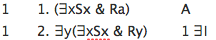In using ∃I you always need to choose a new variable or the expression you write down will not be a well-formed formula.

Explain why "∃x(∃xSx & Rx)" is not a well-formed formula of MPL.

On the list of rules, Rule ∃I is stated precisely using some shorthand symbolism:

#### ∃I (Existential Quantifier Introduction)

For any variable v and name c, if you have derived φv/c, and ∃vφ is a well-formed formula of MPL, then you can write down ∃vφ, depending on everything φv/c depends on.

This statement of the rule uses the symbolism "φv/c". This symbolism is a shorthand which makes it simpler to state the quantifier rules. As we will be using it, "φv/c" means that you take an expression φ and replace all occurrences of v with c. For example, if φ is "(Fx&Fb)", v is "x" and c is "a", then φx/a is "(Fa&Fb)". If v is "x" and c is "b" then φx/b is "(Fb&Fb)".

State Rule ∃I without the shorthand symbolism.

## §3. Rule of Universal Quantifier Elimination

A second straightforward rule for the quantifiers is the rule of universal quantifier elimination, ∀E:

#### ∀E (Universal Quantifier Elimination)

For any variable v and name c, if you have derived ∀vφ, then you can write down φv/c, depending on everything ∀vφ depends on.

This rule is easy to use. For instance: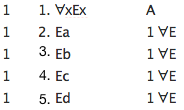Or, another example: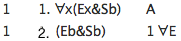However, the following is incorrect: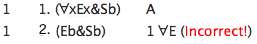This is incorrect because line 1 is a conjunction, not a formula beginning with a universal quantifier. Since the formula on line 1 does not begin with a universal quantifier, ∀E does not apply. ∀E only applies to formulas beginning with a universal quantifier.

Explain why Rule ∀E is a sound rule.

## §4. An example

Here is an example of a derivation in MPL using rules you have learned so far:This shows (Sb→∀xEx), ~Ea ⊢ ~∀xSx .

Find a shorter derivation showing that (Sb→∀xEx), ~Ea ⊢ ~∀xSx .